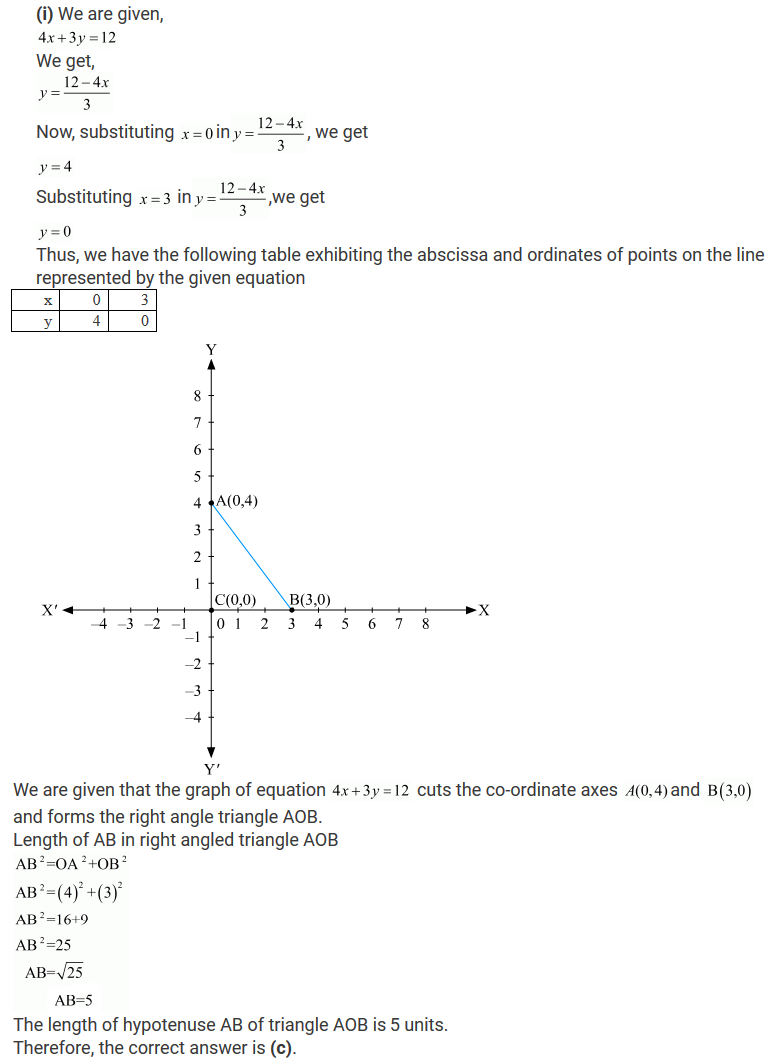#### Chapter 13 Linear Equations in Two Variables R.D. Sharma Solutions for Class 9th MCQ's

Exercise 13.3

Mark the correct alternative in each of the following:

1. If (4, 19) is a solution of the equation y = ax + 3, then a=
(a) 3
(b) 4
(c) 5
(d) 6

Solution

We are given (4,19)as the solution of equation.
y = ax+3
Substituting x= 4 and y= 19, we get
19 = 4a+3
⇒ 4a=19-3
⇒ 4a=16
⇒ a=4
Therefore, the correct answer is (b).

2. If (a, 4) lies on the graph of 3x + y = 10, then the value of a is
(a) 3
(b) 1
(c) 3
(d) 4

Solution

We are given (a, 4) lies on the graph of linear equation 3x+ y= 10.
So, the given co-ordinates are the solution of the equation 3x+ y= 10.
Therefore, we can calculate the value of a by substituting the value of given co-ordinates in equation 3x+ y= 10.
Substituting x= a and y= 4 in equation 3x+ y= 10, we get
3×a + 4 = 10
⇒ 3a = 10-4
⇒ 3a=6
⇒ a=6/3
⇒ a=2
No, option is correct.

3. The graph of the linear equation 2x − y = 4 cuts x- axis at
(a) (2, 0)
(b) (−2, 0)
(c) (0, −4)
(d) (0, 4)

Solution

Given,
2x- y = 4
we get,
y = 2x-4
We will substitute y =0 in y = 2x-4 to get the co-ordinates for the graph of 2x- y = 4 at x axis
0 = 2x-4
⇒ 2x=0+4
⇒ 2x=4 x=2
Co-ordinates for the graph of 2x- y = 4 are (2,0).
Therefore, the correct answer is (a).

4. How many linear equations are satisfied by x = 2  and y = −3?
(a) Only one
(b) Two
(c) Three
(d) Infinitely many

Solution
There are infinite numbers of linear equations that are satisfied by x= 2 and y = -3 as
(i) Every solution of the linear equation represent a point on the line.
(ii) Every point on the line is the solution of the linear equation.
Therefore, the correct answer is (d).

5. The equation x − 2 = 0 on number line is represented by

(a) a line
(b) a point
(c) infinitely many lines
(d) two lines

Solution

The equation x - 2 = 0  is represented by a point on the number line.
Therefore, the correct answer is (b).

6. x = 2, y = −1 is a solution of the linear equation
(a) x + 2y = 0
(b) x + 2y = 4
(c) 2x + y = 0
(d) 2x + y = 5

Solution

We are given x=2; y = -1 as the solution of linear equation, which we have to find.
The equation is x+2y = 0 which can he proved by
Substituting x=2 and y= -1 in the equation x+2y= 0, we get
2 + 2×(-1) = 0
2-2 = 0
0 = 0
RHS = LHS
Therefore, the correct answer is (a).

7. If (2k − 1, k) is a solution of the equation 10x − 9y = 12, then k =
(a) 1
(b) 2
(c) 3
(d) 4

Solution

We are given (2k-1, k)as the solution of equation
10x - 9y = 12
Substituting x=2k-1 and y = k , we get
10 ×(2k-1)- 9×k =12
⇒ 20k - 10-9k =12
⇒ 11k = 12+10
⇒ 11k = 22
⇒ k = 22/11
⇒ k = 2
Therefore, the correct answer is (b).

8. The distance between the graph of the equations x = −3 and x = 2 is
(a) 1
(b) 2
(c) 3
(d) 5

Solution

Distance between the graph of equations x= -3 and x = 2.
D = Distance of co-ordinate on negative side of x axis + Distance of co-ordinate on positive side of x axis
Distance of co-ordinate on negative side of x axis = x = 3 units
Distance of co-ordinate on positive side of x axis = x = 2 units
D = 3+2
D = 5 units
Therefore, the correct answer is d

9. The distance between the graphs of the equations y = −1 and y = 3 is
(a) 2
(b) 4
(c) 3
(d) 1

Solution

Here, you can see, the distance is 4 units. The distance can be calculated by subtracting y coordinates. 3-(-1) =4. So, (b) is the correct answer.

10. If the graph of the equation 4x + 3y = 12 cuts the coordinate axes at A and B, then hypotenuse of right triangle AOB is of length
(a) 4 units
(b) 3 units
(c) 5 units
(d) none of these

Solution